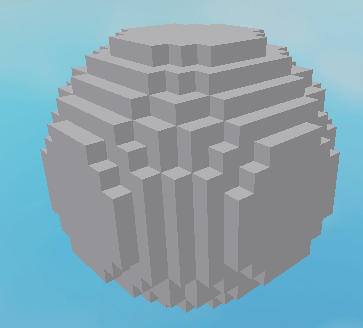# Creating a polygon (sphere) out of an unsorted list of points

Hi! I have a blocky sphere generation and I want to convert it to a sphere out of triangles generator. I already have the code for the triangles which accepts 3 points and makes a triangle. However, how do I find those 3 points in an array of points around the sphere? Is there an algorithm to sort them?

Code I have so far:

``````local ServerStorage = game:GetService("ServerStorage")

local modules = ServerStorage.Modules
local Triangle = require(modules.Triangle)

math.randomseed(tick())

local planet = workspace.Planet

return isBorder
end

local Points = {}

local planetDiamater = planetRadius * 2

local centerCFrame = CFrame.new(position)

for x = 0, planetDiamater do
for z = 0, planetDiamater do
for y = 0, planetDiamater do
local width = planetDiamater * resolution
local offset = CFrame.new(-(width / 2) - resolution / 2, -(width / 2) - resolution / 2, -(width / 2) - resolution / 2)
local blockCF = centerCFrame * CFrame.new(x * resolution, y * resolution, z * resolution)	 * offset

local part = Instance.new("Part")
part.Material = Enum.Material.SmoothPlastic
part.Anchored = true
part.Size = Vector3.new(resolution, resolution, resolution)
part.CFrame = blockCF
part.Parent = planet

table.insert(Points, blockCF.Position)
end
end
end

wait()
end

print("Done generating")
end

generatePlanet(30, Vector3.new(0, 0, 0), 4)
``````1 Like